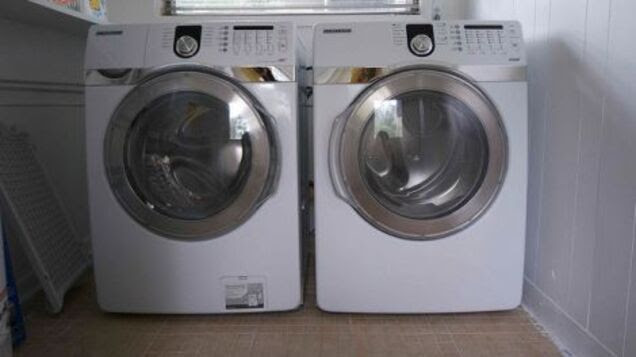### Calculate the Energy Cost of Your Appliances With This FormulaYou can easily find the annual cost estimates of your appliances. But those numbers are based on averages, not your actual usage. Here’s how to calculate a better figure for how much your appliances are running you.
You’ve probably seen those those big yellow labels that tell you how much energy an appliance uses. Financial journalist Liz Weston explains that these aren’t completely accurate, because they’re based on national averages. Your cost might vary quite a bit depending on where you live and how much energy you use.
You could always invest in a usage monitor to get a better idea of your cost. But if you don’t feel like spending the money, here’s a formula that might be more helpful.
First, you need to estimate the appliance’s daily run time. If you run your washer for seven hours a week, for example, that’s one hour a day. Weston explains that refrigerators run about eight hours a day. You also need to know the appliance’s wattage, which can usually be found on the appliance itself. If not, a quick Internet search should yield some results. One last thing: you’ll need to know how much you pay for energy. How many cents do you pay per kilowatt? That info can be found on your electric bill.
Once you have those three bits of info, here’s how to calculate the cost:
1. Calculate the daily energy consumption:
(Wattage) x (hours used per day)
2. Calculate the annual use:(Daily energy consumption) x (number of days the appliance is used per year)
3. Multiply #2 by your energy cost.
I used Weston’s formula to calculate the energy cost of my TV. Here are my results:
1. Daily energy consumption:260 watts X about 4 hours = 1,040
Divided by 1,000= 1.04 kwh of daily consumption
2. Annual use:1.04 X 350 (I shaved some days off for vacation, weekend trips) = 364
3. Multiply by energy cost:
364 x \$0.14 per kwh = \$50.96
My TV costs just over \$50 a year to use. If you want to know where to focus your energy savings, this formula could come in handy. It can also tell you how much you could potentially save by upgrading appliances. For more detail, check out the full post.